## Example Questions

### Example Question #1 : How To Order Fractions From Least To Greatest Or From Greatest To Least

Which fraction is the SMALLEST?

35/50

1/2

4/9

47/100

6/11

4/9

Explanation:

estimate or calculate decimals after simplifying

### Example Question #171 : Fractions

8/9 > x > 6/7

Quantity A: x

Quantity B: 7/8

Quantity B is greater.

The two quantities are equal.

The relationship cannot be determined from the information given.

Quantity A is greater.

The relationship cannot be determined from the information given.

Explanation:

At first glance, it appears that both values are equal.7/8 and x will be between 6/7 and 8/9.

However do a quick calculation on the GRE calculator to figure out that 6/7 is approximately 0.857 and and 8/9 is approximately 0.889. 7/8 (Quantity B) is 0.875. x could equal 0.86 and be less than Quantity B or x could equal 0.88 and be greater than Quantity B. Because at least two possibilities exist (x could also equal 7/8), there is not enough information given to determine the relationship.

### Example Question #172 : Fractions

Which of the following fractions is the greatest?

1/2

15/21

8/11

17/23

19/25

19/25

Explanation:

The trick here is to set 1/2 as a baseline; all other choices are greater than 1/2.

Next is to see how much the difference is between each numerator and denominator (if you multiply 8/11 by 2/2, it will become 16/22) - each fraction besides 1/2 has a numerator that is 6 units less than the denominator. The trick is that the largest numerator will be the largest fraction when the numerator and denominator are the same units apart on all fractions.

### Example Question #1 : Ordering

Which is greater,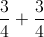or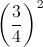?

The relationship cannot be determined from the information given.is greater

The quantities are equal.is greateris greater

Explanation:

To add fractions with the same denominator, add their numerators and leave the denominator the same: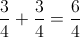To square a fraction, square its numerator and denominator: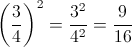For comparison, rewrite the first fraction with 16 as its denominator also: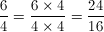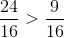so### Example Question #174 : Fractions

Quantitative Comparison

Quantity A: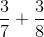Quantity B: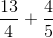The two quantities are equal.

Quantity A is greater.

Quantity B is greater.

The relationship cannot be determined form the information given.

Quantity B is greater.

Explanation:

One option is to do the actual math to find the answer. Quantity A = 3/7 + 3/8 = 45/56. Quantity B = 13/14 + 4/5 = 121/70. Here Quantity A < 1 and Quantity B > 1, so it is easy to tell which is bigger. This isn't always the case however, so often doing the math is NOT the quickest or easiest way to solve GRE questions. For example, if the two answers had been 45/56 and 72/83, it would have been very difficult to tell which was the larger fraction just by looking at them.

Instead of computationally figuring this question out, we can break it into parts.

Let's look at the first fraction in the two columns: 3/7 < 1/2 and 13/14 > 1/2, so the first fraction in Quantity B is bigger.

Next look at the second fraction:  3/8 < 1/2 and 4/5 > 1/2, so the second fraction in Quantity B is bigger.

Now we know that Quantity B is bigger without having to do any real math!

### Example Question #581 : Arithmetic

Which of these fractions is the LARGEST?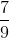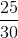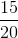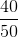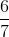Explanation:

Now that the GRE has a built-in calculator, simply find the decimal equivalent of each of the answer choices to see which is the largest!

### Example Question #1 : How To Order Fractions From Least To Greatest Or From Greatest To Least

Which of the following is the greatest fraction: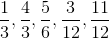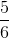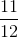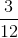Since the fractionis the only one with a numerator larger than its denominator, it is the only one greater than one.  That means it is the largest fraction.